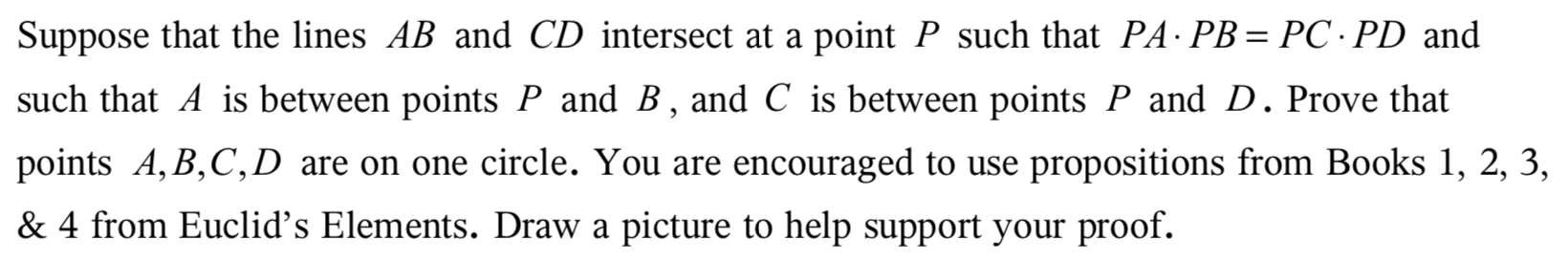Suppose that the lines AB and CD intersect at a point P such that PA PB PC PD andsuch that A is between points P and B, and C is between points P and D. Prove thatpoints A, B,C,D are on one circle. You are encouraged to usepropositions from Books 1, 2, 3,& 4 from Euclid's Elements. Draw apicture to help support your proof.

Questionhelp_outlineImage TranscriptioncloseSuppose that the lines AB and CD intersect at a point P such that PA PB PC PD and such that A is between points P and B, and C is between points P and D. Prove that points A, B,C,D are on one circle. You are encouraged to use propositions from Books 1, 2, 3, & 4 from Euclid's Elements. Draw a picture to help support your proof. fullscreen
Step 1

Given that the lines AB and CD intersects at a point P such that

Step 2

Since, there is a theorem that, “A circle always passes through three non-collinear points.”

So, draw the circle through three non-collinear points A, B and C and suppose that point D is not on the circle.

Then two cases are arising,

Case 1: When the point D is outside the circle, then the line CD intersects the circle at D’ as shown in the following diagram,

Step 3

By the diagram AP.PB=CP.PD’

But given that PA.PB=PC.PD

Then supposition t...

Want to see the full answer?

See Solution

Want to see this answer and more?

Our solutions are written by experts, many with advanced degrees, and available 24/7

See Solution
Tagged in

Math